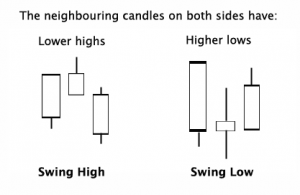Select Page

Fibonacci retracements in forex and other forms of trading using technical analysis. This is a fascinating subject. Leonardo Fibonacci was a 13th Century mathematician. He was considered by many scholars to have been one of the most important and influential of the middle ages.Using data from Hindu-Arabic numerals he created a sequence of numbers that became more famously known as the 61.8%, golden ratio.

This sequence of numbers is still used to this day in sophisticated trading algorithms by technical traders from all over the world.

# Fibonacci Retracement

Fibonacci retracement is a method of calculation used widely by forex & commodity traders.

The Fibonacci sequence first appeared as the solution to a problem in the Liber Abaci a book written by Leonardo Fibonacci in 1202 to introduce the Hindu-Arabic numerals used today to a Europe still using Roman numerals.

Basically, Fibonacci numbers are resultant from adding the previous together to find the next. For example : 1+1=2, 2+1=3, 3+2=5, 5+3=8 and so on. The Fibonacci string would look like: 0, 1, 1, 2, 3, 5, 8, 13, 21, 34, 55, 89, 144, 233…

### Fibonnacci Retracement

Fibonacci retracement & the “golden ratio.” After the first few numbers in the Fibonacci sequence, the ratio of any number to the next higher number is approximately .618, and the lower number is 1.618. These two figures are the golden mean or the golden ratio.

Fibonaccis golden ratio formula appears throughout biology, art, music, and architecture. For example DNA molecules, sunflowers, snail shells, galaxies, and hurricanes. Nowadays the method is widely used in forex trading.

## Fibonacci Retracement

The Fibonacci retracement methods and formulas are widely used to calculate trades. However all the miraculous maths in the world will never make Fibonacci retracement true 100% of the time. Therefore a little common sense goes a long way when using any type of mathematical or technical indicator to trade from.

Fibonacci retracement is only good so long as enough people are watching and acting on the same information that the collective whole makes the occurrence a self-fulfilling forecast.

Basically put, even if you’ve opened a trade based on Fibonacci retracement expectations, be prepared to close the position, should common sense warrant you to. If you are now worrying that your maths isn’t up to scratch, don’t worry. There are many free online calculators which will do the hard work. You just need to understand the basics and the method behind it.

## How Traders Use Fibonacci Retracement

Traders use the Fibonacci retracement levels as potential support and resistance areas. Fibonacci Retracement is the collapsing of price when it hits a certain level. If the price is moving in the Fibonacci patterns, you will find that it will be supported by the 0.382, 0.5 or the 0.618 levels and this is usually where you can take your trades waiting for reversal.

Traders use the Fibonacci extension levels as profit taking levels. Again, since so many traders are watching these levels to place buy and sell orders to take profits, these tool tends to work more often than not. Most charting software includes both Fibonacci retracement levels and extension level tools. In order to apply Fibonacci levels to your charts, you’ll need to identify Swing High and Swing Low points.A Swing High is a candlestick with at least two lower highs on both the left and right of itself.

A Swing Low is a candlestick with at least two higher lows on both the left and right of itself.

### Fibonacci Retracement -how to actually trade

Step 1: Draw a Fibonacci retracement level (use the tools in metatrader 4 to do this automatically)

Step 2: Check if there is any support on the 0.382, 0.5 , 0.618 or 78.6% fibonacci levels

Repeat step 1 until you can find one. If there is support formed on any of the levels in step 2, you can then look for opportunity to get into a trade and then pick the extension level as your exit position.

Take a look at the video below where I show the Fibonacci retracement method in more detail. If you would like to learn more about how to use fibonacci for entries, exits, stops and targets then consider joining my low cost forex mentor program.

If you would like to learn how to trade like a professional check out our 5* rated forex mentor program, RISK FREE; by clicking on the “Get Started Today” Button below

To find out more thoughts and inside tips on forex trading, take a look at some of our blogs.